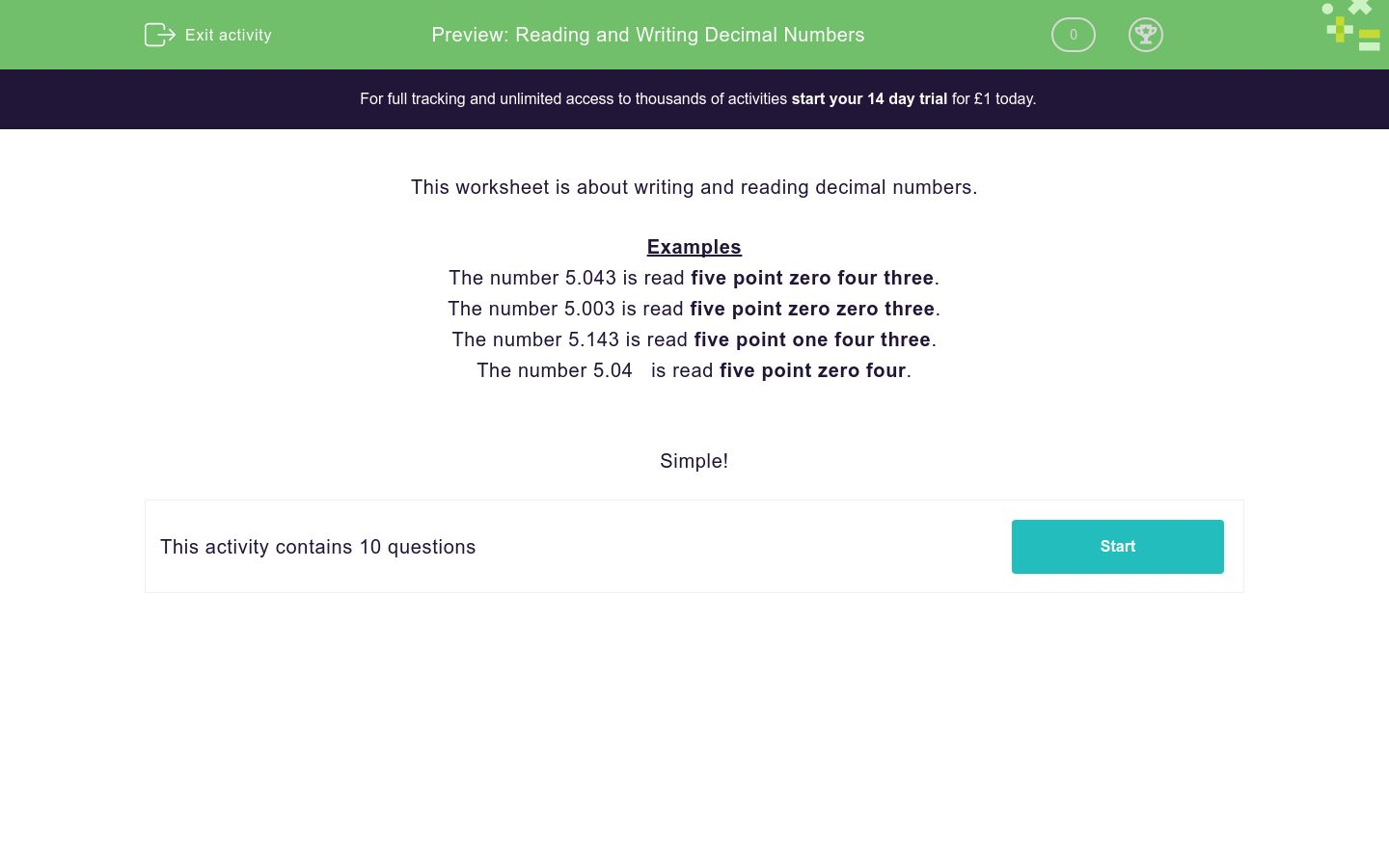# Reading and Writing Decimal Numbers

In this worksheet, students revise and practise reading and writing simple decimal numbers.Key stage:  KS 3

Curriculum topic:   Number

Curriculum subtopic:   Understand Order for All Numbers

Difficulty level:### QUESTION 1 of 10

Examples

The number 5.043 is read five point zero four three.

The number 5.003 is read five point zero zero three.

The number 5.143 is read five point one four three.

The number 5.04   is read five point zero four.

Simple!

---- OR ----